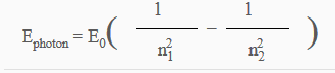# Equation for modeling atomic spectra of all atoms

• I
• Xilus

#### Xilus

I've seen the equation I think is just for hydrogen. is this just for hydrogen?of course this doesn't return the atomic spectra, it returns the energy.
So using E=h*v and Planck's constant. a simple factor of 1/h would return the frequency.
right? Energy is directly proportional to frequency.
and E0=13.6eV n1<n2 where both n1 and n2 are integers

Is there an equation that models atomic spectra of all atoms?

is this just for hydrogen?
Yes it's only for hydrogen, and approximately for the so-called Rydberg states.

So using E=h*v and Planck's constant. a simple factor of 1/h would return the frequency.
right?
Yes.

Is there an equation that models atomic spectra of all atoms?
As far as I know, no. We haven't derived the general expression for energy levels for all atoms.

is the spectra the same for all isotopes?

is the spectra the same for all isotopes?
There is the so-called isotope shift which arise due to the fact that the nucleus is not completely at rest. It moves around by a very little amount which in turn disturbs the motion and hence wavefunction and energy levels of electrons. Different nuclear mass will have different effect on the wavefunction.

The formula for the isotope shift is quite simple. It's just a scaling by the reduced mass.
The energy is
$$E_M = \frac{M}{m_e+M} E_\infty \left(\frac{1}{n_1^2} - \frac{1}{n_2^2}\right)$$
where ##m_e## is the electron mass, ##M## is the nuclear mass, and ##E_\infty \approx 13.605693## eV

I think quantum mechanics brings in probabilities into physics ,so due to no determinism we cannot describe a general result for multielectron atoms.

That's not the reason we don't have a general result. The reason is that it's just too complicated for a simple analytical formula.